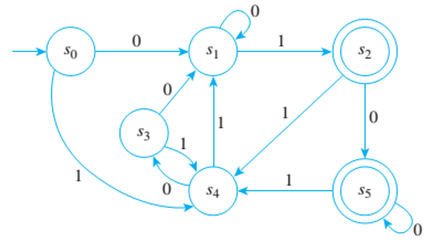Chapter 12.3, Problem 5ES### Discrete Mathematics With Applicat...

5th Edition
EPP + 1 other
ISBN: 9781337694193

#### Solutions

Chapter
Section### Discrete Mathematics With Applicat...

5th Edition
EPP + 1 other
ISBN: 9781337694193
Textbook Problem
1 views

# Consider the finite-state automaton given by the following transition diagram:a. Find the 0-, 1-, 2-, and 3-equivalence classes of states of A. b. Draw the transition diagram for A ¯ , the quotient automaton of A.

To determine

(a)

Find the 0,1,2and 3 equivalence classes of states of A.

Explanation

Given information:

Concept used:

Arrow in the given graph played vital role in answer selection.

Calculation:

It is required to find the 0,1,2and 3 equivalence classes of states of A.

0 Equivalence classes- Two states are zero equivalent if both are accepting states or both are

non-accepting states. Therefore, the 0 equivalence classes are: {s0,s1,s3,s4},{s2,s5}

1 Equivalence classes- Two states are 1 equivalent if and only if they are

0 equivalent and after input of any symbol, their next state are 0 equivalent. Therefore, the 1 equivalence classes are: {s0,s3,s4},{s2,s5},{s1}

2 Equivalence classes- Two states are called 2 equivalent if and only if they are 1 equivalent and after input of any symbol, their next state are 1 equivalent. Therefore, the 2 equivalent classes are: {s5,s2},{s1},{s3},{s4},{s0}

3 Equivalence classes- Two states are called 3 equivalent if and only if they are 2 equivalent and after input of any symbol, their next state are 2 equivalent

To determine

(b)

Draw the transition diagram for A¯, the quotient automaton of A.

### Still sussing out bartleby?

Check out a sample textbook solution.

See a sample solution

#### The Solution to Your Study Problems

Bartleby provides explanations to thousands of textbook problems written by our experts, many with advanced degrees!

Get Started